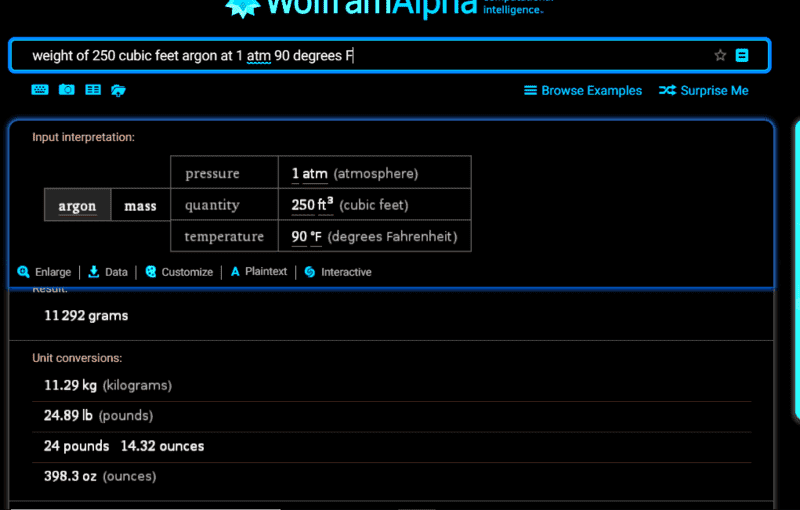# Cubic Feet of Argon required to fill a tank under vacuum

Nathan Smith
I have a tank that is 250 Cubic Feet.
The tank is under vacuum (1x10-5 Torr).. which is actually 1.93368e-7 psia.
How much Argon, in cubic feet, does it take to back-fill to atmosphere or 14.7 psia?
Ambient temperature in tank is 90 degrees.

Homework Helper
I have a tank that is 250 Cubic Feet.
The tank is under vacuum (1x10-5 Torr).. which is actually 1.93368e-7 psia.
How much Argon, in cubic feet, does it take to back-fill to atmosphere or 14.7 psia?
Ambient temperature in tank is 90 degrees.
Hello Nathan,I don't suppose you mean 250 cubic feet ? But then: what do you mean ?

Nathan Smith
Is it that simple from a vacuum?

I have a tank that is 250 Cubic Feet.
The tank is under vacuum (1x10-5 Torr).. which is actually 1.93368e-7 psia.
How much Argon, in cubic feet, does it take to back-fill to atmosphere or 14.7 psia?
Ambient temperature in tank is 90 degrees.

Well, it will take about 250 cubic feet. Gas volumes are normally quoted at STP (standard temperature and pressure, which is 0 degrees C and 1 atm). You want 1 atm of pressure and 90 degrees. I'm going to assume, since your other measurements are in English units, that you mean 90 degrees F, which is 305 degrees K. So the volume of the gas will increase by 305/273, so you will need somewhat less than 250 cu ft of gas. You will need 250*273/305 = 224 cu ft of Ar to fill your tank. So one large gas cylinder will do it.

Staff Emeritus
Pretend that the initial vacuum was perfect. Then your question is how much argon does it take to occupy 250 cubic feet at 90 degrees F?

The answer can not be given in cubit feet, because we don't know the pressure of the argon before it was put into this tank. You may be getting the argon from another tank at 1000 psi.

Wolfram alpha is a good source for such questions.Now your next question is how many cubic feet is 25 pounds of argon? You have to tell me the pressure to answer that.

#### Attachments

Nathan Smith
The source 130 PSI supply pressure from a 16 pack of Argon cylinders with 5721 cubic feet of gas storage.

edwould
It's been too long since I did this stuff but the Ideal Gas Law is used to determine how much volume an IDEAL gas occupies at different temperatures and pressures
https://www.engineeringtoolbox.com/ideal-gas-law-d_157.html

and the van der Waals equation is used to adjust for the fact that different gases behave differently and thus DEVIATE from the IDEAL equation's results. The following link explains it and has data for 200 or so different gases, including Argon.

https://www.engineeringtoolbox.com/...-Waals-equation-constants-gas-law-d_1969.html

Hope this helps. I'm not a physicist, just remember some of this from my brief stint in engineering school roughly 1000 years ago.

Gold Member
how many cubic feet is 25 pounds of argon?

Tell me how big your piece of string tank is and I will tell you how big the argon becomes. At least for reasonable sized tanks.

BoB